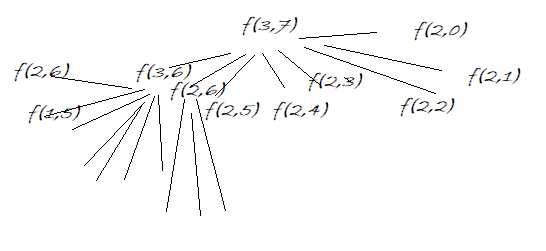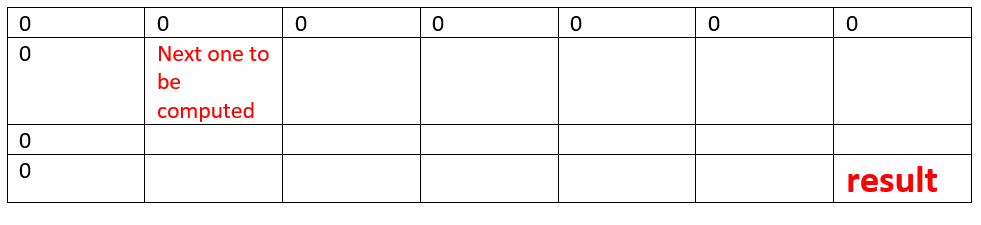# Maximum Profit in Stock Buy and sell with at most K Transaction

Here, we are going to learn about Maximum Profit in Stock Buy and sell with at most K Transaction using dynamic programming.
Submitted by Radib Kar, on January 05, 2020

This is a very popular interview problem to find maximum profit in stock buying and selling with at most K transactions. This problem has been featured in the interview rounds of Amazon.

Problem statement:

In stock market, a person buys a stock and sells it on some future date. Given the stock prices of N days in form of an array Amount and a positive integer K, find out the maximum profit a person can make in at most K transactions. A transaction is buying the stock on some day and selling the stock at some other future day and new transaction can start only when the previous transaction has been completed.

```    Input:
K=3
N=7

Stock prices on N days:
10 25 38 40 45 5 58

Output:
88
```

Example:

```    Number of maximum transactions: 3
Total number of days, N: 7

To achieve maximum profit:
Stock bought at day1    (-10)
Stock sold at day5      (+45)
Stock bought at day6    (-5)
Stock sold at day7      (+58)

Total profit = 88
```

Explanation:

Let there are N number of days for transactions, say x1, x2, ..., xn

Now for any day xi

1. Don't do any transaction on the day xi
2. Transact on day xi, i.e., buy stock on some day xj and sell on day xi where j<i and i,j Є N

Total number of transactions can be made at most = K

Now we can formulate the maximum profit case using above two condition.

Let,

```    f(t,i)=maximum profit upto ith day and t transactions
```

Considering the above two facts about xi

```    f(t,i)=f(t,i-1)  if there is no transaction made on day xi ...(1)
Max(f(t-1,j)+amount[i]-amount[j])
where j<i and i,j Є N if there is transaction  on day xi ...(2)
Obviously, to maximize the profit we would take the maximum of (1)  and (2)

Number of maximum transactions: 3
Total number of days, N: 7
Stock prices on the days are:
10 25 38 40 45 5 58
```

Below is a part of recursion tree which can show that how many overlapping sub problems there will be.Figure 1: Partial recursion tree to show overlapping sub-problems

So we need dynamic programming...

Problem Solution

Recursive Algorithm:

```    Function(t, i): //f(t, i)
If i=0
f(t, i)=0
If t=0
f(t, i)=0

f(t, i)= f(t, i-1); //no transaction on day xi
For j =0: i
Find max(f(t-1,j) + amount(i)- amount(j)
End For
If the maximum found > f(t, i)
update f(t, i)
End IF
Return f(t, i)
```

Conversion to DP

```    For tabulation we need a 2D array, DP[k+1][n] to store f(t,i)

Base case,
for i 0 to k,   DP[i]=0 //no profit on 0th day
for i 0 to n-1,   DP[j]=0 //no profit on 0 transaction

To fill the higher values,
for t=1 to k
for i =1 to n-1
DP[t][i]=DP[t][i-1]
for j= 0 to i-1 //buying on jth day and selling on ith day
DP[t][i]=max(DP[t][i],DP[t-1][j]+ amount[i] –amount[j])
End for
End for
End for
Result would be f(k,n) that is value of DP[k][n-1]
```

Initial DP table

```    DP //K=3, N=7
```Try yourself to compute the DP table manually following the above algorithm and find out the result. Take some small example if necessary.

C++ implementation:

```#include <bits/stdc++.h>
using namespace std;

int Max_profit(vector<int> amount, int n, int k)
{
int DP[k + 1][n];
memset(DP, 0, sizeof(DP));

//on 0th day
for (int i = 0; i <= k; i++)
DP[i] = 0;
for (int i = 0; i <= n; i++)
DP[i] = 0;

for (int t = 1; t <= k; t++) {
for (int i = 1; i < n; i++) {
DP[t][i] = DP[t][i - 1];
int maxV = INT_MIN;
//buying on jth day and selling on ith day
for (int j = 0; j < i; j++) {

if (maxV < DP[t - 1][j] + amount[i] - amount[j])
maxV = DP[t - 1][j] + amount[i] - amount[j];
}
if (DP[t][i] < maxV)
DP[t][i] = maxV;
}
}
return DP[k][n - 1];
}

int main()
{
int n, item, k;

cout << "Enter maximum no of transactions:\n";
cin >> k;
cout << "Enter number of days\n";
cin >> n;
vector<int> amount;
cout << "Enter stock values on corresponding days\n";
for (int j = 0; j < n; j++) {
scanf("%d", &item);
amount.push_back(item);
}

cout << "Maximum profit that can be achieved: " << Max_profit(amount, n, k) << endl;

return 0;
}
```

Output

```Enter maximum no of transactions:
3
Enter number of days
7
Enter stock values on corresponding days
10 25 38 40 45 5 58
Maximum profit that can be achieved: 88
```